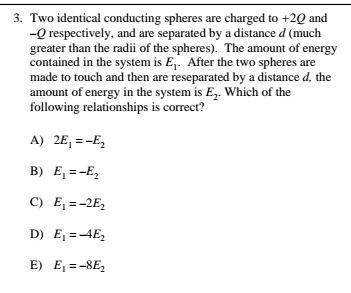# Energy in system of two conducting spheres

• RoboNerd

#### RoboNerd

1. Homework StatementHi everyone. I am having trouble figuring out how to solve this problem. The right answer is E

## Homework Equations

Well, we do have the law of conservation of charge.
I also know that Work by a conservative force = - change in potential energy

## The Attempt at a Solution

So I am having trouble figuring out a general approach.
First things first, when I have two of these spheres touch, they are going to become equipotential spheres. I thus have a charge of 0.5*Q on each of them. Then I said that one of these spheres is fixed and we are moving the other one out and out while the electric field from the first is doing work on it.

This could change its electric potential energy.

How is my approach so far?

Thanks in advance for the help and being willing to help.

You are almost there! Have you been taught an expression for potential energy between charges? How about an expression for force that you could integrate?

Work by a conservative force = - change in potential energy

No need to worry about forces here, this is all about energy. Forces would get very messy over the range where the spheres are close.
What is the initial PE in terms of Q and d?

Well... I do remember that electric potential is going to be equal to the negative integral of the electric field (bringing in a force from infinity to its final position)

Have you been taught an expression for potential energy between charges?
Voltage = - (Integral) [E dot dl]
Yes.

I think that the electric field could be derived for one of the spheres, and then saying that the second sphere is like that point charge that is brought from infinity to the final position. The problem is that the point charge (or this sphere) is not being brought in from infinity to its final position but rather pulled away from the first sphere to its final position.

What is the initial PE in terms of Q and d?
In response to this, I would say that the initial potential energy for each of the spheres is going to be equal to:

1/(4*pi*e0) * charge on it / radius sphere^2

The problem with this approach is that the radius of the sphere is not "d."

As you may see I am getting a little confused. Please advise me on how to proceed. Thanks for being willing to help, and make it a great day!

the initial potential energy for each of the spheres
You are told that d is much greater than the radius of the spheres. That means the PE of the pair of spheres, in regard to their attraction to each other, dwarfs the PEs of the individual spheres. I.e., treat them as point particles.

•RoboNerd
Thanks for the input.

Here is what I did now.

For the initial situation where one sphere has -Q and the other +2Q charge, I made one charge stationary, and then I did the integration of E dot dl to get its potential.
Then I multiplied it by the charge of the stationary sphere as per the relationship U = q * Voltage

Thus for Initial Situation:

U = q*V = 2Q * [negative integral from infinity to d of E dot dl].
The electric field E in this case is -Q/(4*pi*e0*r^2).

Doing this gave me Energy #1 = -2*Q^2/(4*pi*e0*d)

OK... now for the second situation where each sphere has a 1/2*Q charge. Same approach.

U = q*V = (1/2)*Q * [negative integral from infinity to d of E dot dl].
The electric field E in this case is (1/2)*Q/(4*pi*e0*r^2).

Doing this gave me
Energy #2= (0.25) * Q^2 / (4*pi*e0*d)

OK. Setting energies #1 and #2 in both of these in a ration of E1 : E1 gives me -8 : 1.

Very similar to the right answer, but in the right answer, the numbers in the ration are 1 : -8, opposite of what I have.

What did I do wrong here?
Thanks for being willing to help.